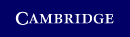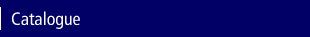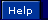Home > Catalogue > Dynamic Modeling and Control of Engineering SystemsSearch this book

Resources and solutions

This title has free online support material available.

Details

• Page extent: 0 pages

(ISBN-13: 9780511287428)

• Also available in Paperback | Hardback
• For price and ordering options, inspection copy requests, and reading lists please select:

Please note local prices may apply

This textbook is ideal for a course in engineering systems dynamics and controls. The work is a comprehensive treatment of the analysis of lumped parameter physical systems. Starting with a discussion of mathematical models in general, and ordinary differential equations, the book covers input/output and state space models, computer simulation and modeling methods and techniques in mechanical, electrical, thermal and fluid domains. Frequency domain methods, transfer functions and frequency response are covered in detail. The book concludes with a treatment of stability, feedback control (PID, lead-lag, root locus) and an introduction to discrete time systems. This new edition features many new and expanded sections on such topics as: solving stiff systems, operational amplifiers, electrohydraulic servovalves, using Matlab with transfer functions, using Matlab with frequency response, Matlab tutorial and an expanded Simulink tutorial. The work has 40% more end-of-chapter exercises and 30% more examples.

• Provides an integrated approach to modeling across physical domains • Includes extensive tutorials for Matlab and Simulink • Well suited to be used in conjunction with a practical lab class • The new third edition contains 40% more exercises and 30% more examples

Contents

Preface; 1. Introduction; 2. Mechanical systems; 3. Mathematical models; 4. Analytical solutions of system input-output equations; 5. Numerical solutions of ordinary differential equations; 6. Simulation of dynamic systems; 7. Electrical systems; 8. Thermal systems; 9. Fluid systems; 10. Mixed systems; 11. Transfer functions; 12. Frequency analysis; 13. Closed-loop systems and system stability; 14. Control systems; 15. Analysis of discrete-time systems; 16. Digital control systems; Appendix 1. Fourier series and the Fourier transformation; Appendix 2. Laplace transformations; Appendix 3. Matlab tutorial; Appendix 4. Simulink tutorial; Index.